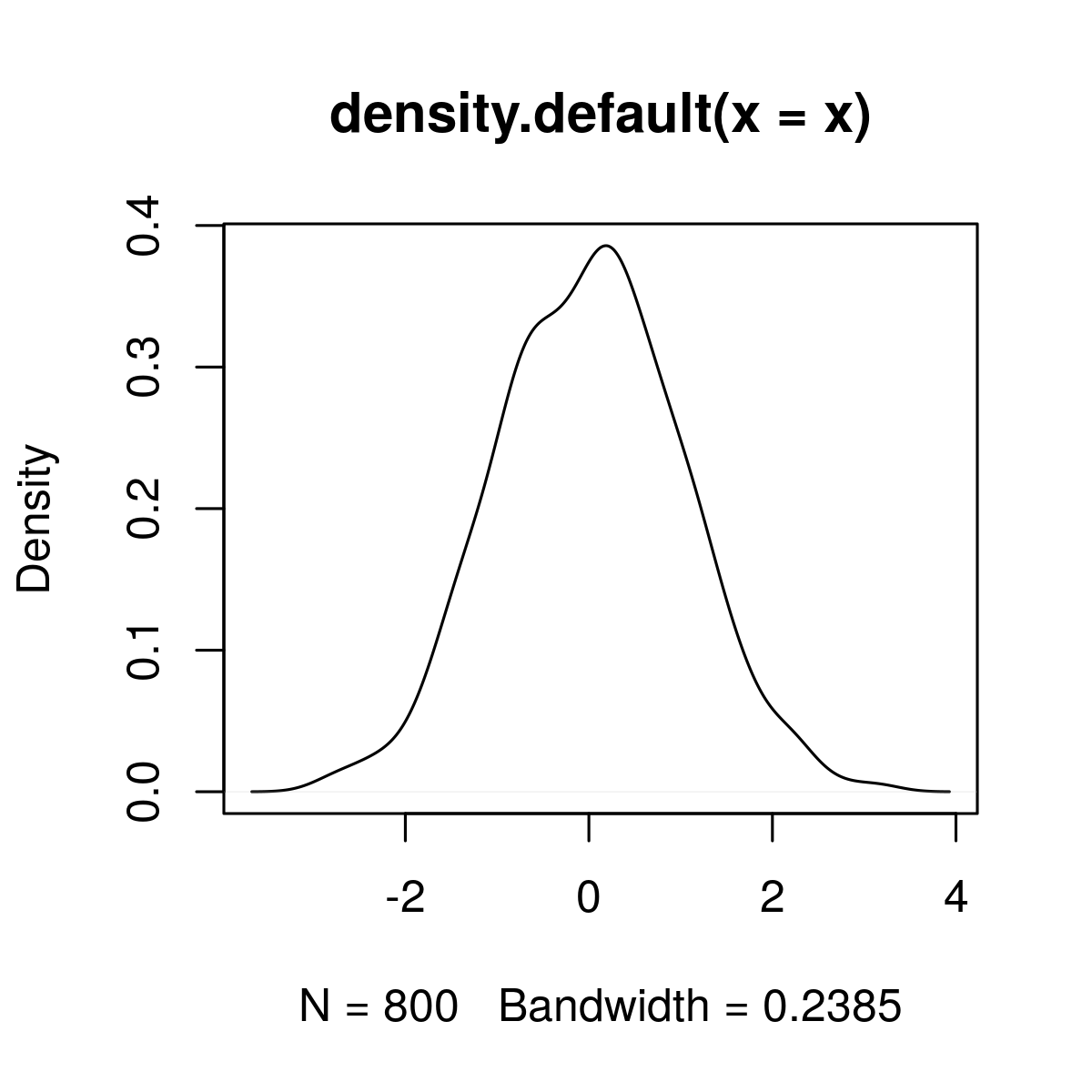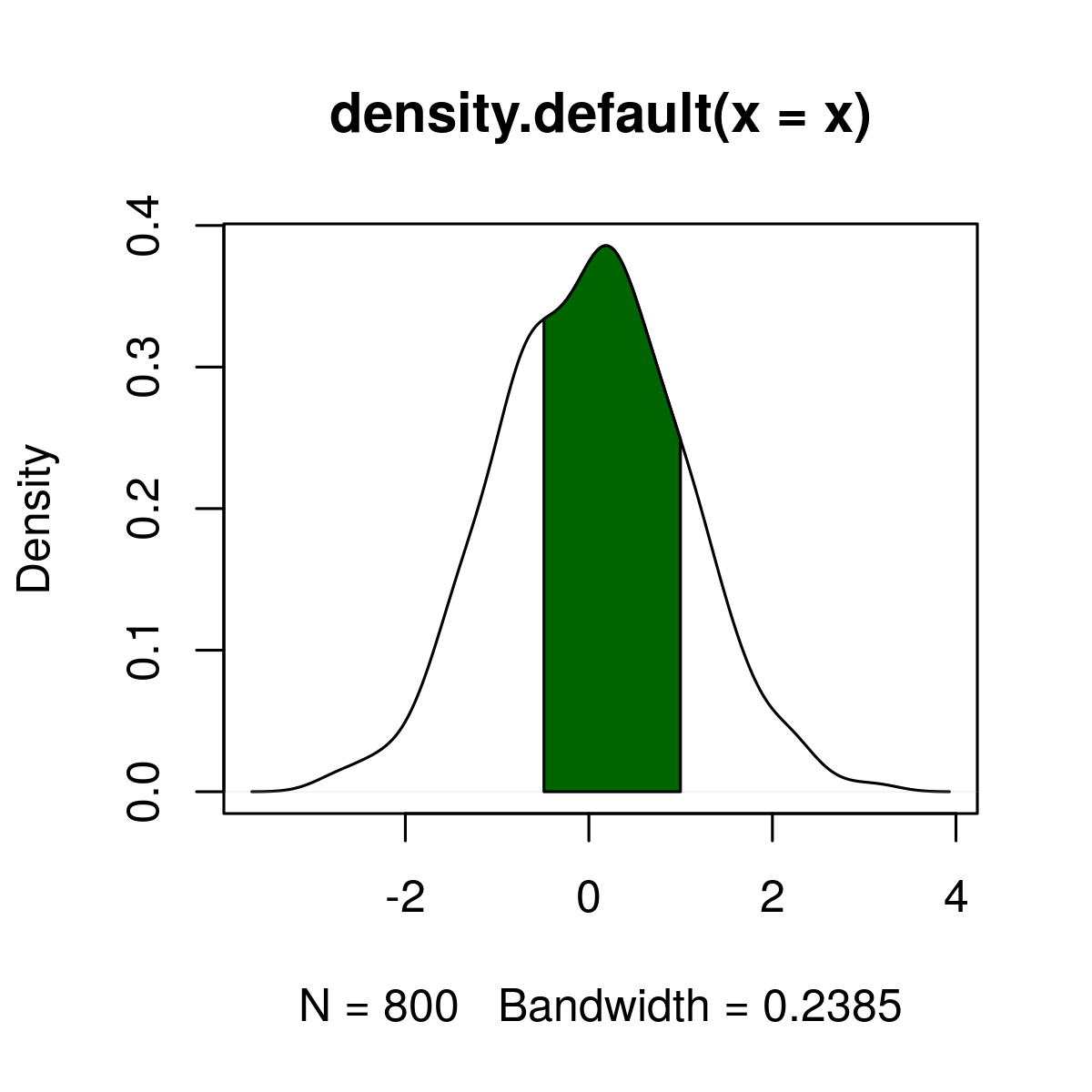# Add Color Between Two Points of Kernel Density Plot in R Programming – Using with() Function

plot() and with() functions are used to draw and add color between a particular area of two points of Kernel Density Plot in R Language.

#### Draw a Kernel Density plot

`plot()` function in R Language is used to draw a Kerner Density Plot

Syntax: plot(dens_x)

Parameters:
dens_x: Default plot of density.

Returns: Kernel density plot

 `# Create example data ` ` `  `set.seed``(72500)   ` `x <- ``rnorm``(800) ` `dens_x <- ``density``(x) ` `plot``(dens_x)        `

Output:`with()` function is used to add color between two points of the graph.

Syntax:
with(dens_x,
polygon(x = c(x),
y = c(0, y[x_low:x_high], 0),
col = ” “))

Parameters:
dens_x: Default plot of density.
x_low, x_high: lower limit and upper limit of colored area
col: Name of color

Returns: Color plot between to particular plots with specified color.

 `# color the plot ` ` `  `set.seed``(72500)   ` `x <- ``rnorm``(800) ` `dens_x <- ``density``(x) ` `plot``(dens_x)        ` `x_low <- ``min``(``which``(dens_x\$x >= - 0.5))   ``# Define lower limit of colored area ` `x_high <- ``max``(``which``(dens_x\$x < 1))       ``# Define upper limit of colored area ` `with``(dens_x,                             ``# Add color between two limits ` `     ``polygon``(x = ``c``(x[``c``(x_low, x_low:x_high, x_high)]), ` `             ``y = ``c``(0, y[x_low:x_high], 0), ` `             ``col = ``"darkgreen"``)) `

Output:My Personal Notes arrow_drop_upCheck out this Author's contributed articles.

If you like GeeksforGeeks and would like to contribute, you can also write an article using contribute.geeksforgeeks.org or mail your article to contribute@geeksforgeeks.org. See your article appearing on the GeeksforGeeks main page and help other Geeks.

Please Improve this article if you find anything incorrect by clicking on the "Improve Article" button below.

Article Tags :

Be the First to upvote.

Please write to us at contribute@geeksforgeeks.org to report any issue with the above content.